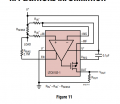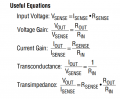# LTC6102 Current sense amplifier calculations

#### zazas321

Joined Nov 29, 2015
926Hey. I am trying to understand this current sensing chip and how it works. I have got an old board with this chip basically the same circuit as shown in an example. I know all Resistor values but I still cannot calculate Vout ir IoutIn my case, Rout = 11kR
Rin = 330R
Rsense = 50mR
V+ (supply voltage) = 26V

From the formulas : Vout = Iout * Rout ( cannot calculate because dont know either whats Vout or whats Iout
Iout = Vsense / Rin (cannot calculate because dont know whats Vsense)

I have been reading through documentation and cant seem to find a solution. There must be a way to determine Vout ir Vsense otherwise I cannot proceed with the calculations. Can someone please give me a hand? Thanks

#### crutschow

Joined Mar 14, 2008
28,208
Vsense is the voltage generated by the load current through Rsense.
Since the op amp tries to keep the differential input voltage equal to zero, it adjusts the MOSFET gate voltage so that the current through the MOSFET and thus Rin- equals Vsense/Rin.
The output voltage is then Vout = Rout * Vsense/Rin.
Since Vsense = Iload * Rsense then
Vout = Iload * Rsense * Rout / Rin.

#### zazas321

Joined Nov 29, 2015
926
Okay that is more clear but do I must know what is going to be my load current before designing this circuit? What if I dont know what is going to be my load current

#### crutschow

Joined Mar 14, 2008
28,208
What if I dont know what is going to be my load current
Since the purpose of the circuit is to measure current then, if you don't know the current, you can't design the circuit.
It's fundamental to any circuit that, if you don't know the requirements, how can you do the design?

Last edited:

#### zazas321

Joined Nov 29, 2015
926
Ok so lets say I connect like a motor or something for Load and I approximately know that its going to draw 1A of current so I design for ILoad 1A>?

#### crutschow

Joined Mar 14, 2008
28,208
Ok so lets say I connect like a motor or something for Load and I approximately know that its going to draw 1A of current so I design for ILoad 1A>?
That's like correct, if 1A is the maximum current you want to measure.